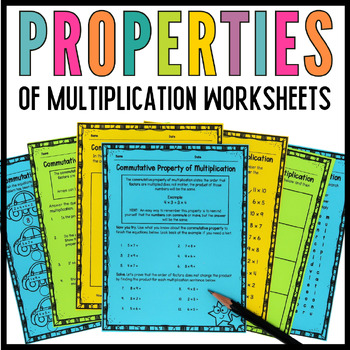DID YOU KNOW:
Seamlessly assign resources as digital activities

Learn how in 5 minutes with a tutorial resource. Try it Now

Learn More# Properties of Multiplication Worksheets 3rd Grade Bundle3rd - 4th, Homeschool
Subjects
Standards
Resource Type
Formats Included
• Zip
• Compatible withActivities
Pages
26 pages
\$6.00
Bundle
List Price:
\$9.50
You Save:
\$3.50
\$6.00
Bundle
List Price:
\$9.50
You Save:
\$3.50
Compatible with Easel Activities
This resource contains one or more resources that are compatible with Easel by TpT, a suite of digital tools you can use to make any lesson interactive and device-ready. Customize these activities and assign them to students, all from Easel. Easel is free to use! Learn more.

### Description

These properties of multiplication worksheets the commutative property, associative property, and distributive property of multiplication break down the properties to your third-grade math students in an engaging way. Your students will learn strategies to solve more complex multiplication sentences and number sentences. The worksheets are also great for practice.

《《This resource is in the process of being 》》

Commutative has been updated.

What's included in the Associative Property of Multiplication:

• Worksheet 1 (copied in pink): Helps your students with the definition and how to recognize the associative property of multiplication.
• Worksheet 2 (copied in orange): A matching worksheet where students will draw a line to the matching number sentence.
• Worksheet 3 (copied in yellow): Allows students to fill in the missing factors in the number sentence.

What's included in the Commutative Property of Multiplication:

• 2-learning the commutative property using arrays worksheets.
• 1-commutative property color by number worksheet (bubble gum machine)f
• 1-worksheet that introduces the definition of the commutative property and breaks it down for understanding.
• 1-finish the equation worksheet (cars)
• 1-matching worksheet
• 1-critical thinking exercise to sharpen those systematic skills.
• 1-word search worksheet introduces your, students, to the vocabulary used in the unit
• 16-task cards students will fill in the missing factor
• 1-recording sheet for task cards

What's Included in the Distributive Property of Multiplication:

• Worksheet 1 (printed in yellow): This worksheet provides the definition of the distributive property of multiplication as well as step-by-step instruction.
• Worksheet 2 (printed in blue): Practice and/or reinforcement of students that struggle "breaking apart" equations.
• Worksheet 3 (printed in pink): Students can practice and refresh their files while practicing the steps of the distributive property of multiplication.
• Worksheet 4 (printed in orange): Word problems for the distributive property of multiplication.
• Worksheet 5 (printed in green): This worksheet can be given at the beginning of the unit. It covers the vocabulary needed to complete the distributive property of multiplication unit. It is encouraging that you make sure students understand the meaning of the vocabulary before beginning the unit.

You may also find these useful:

This resource covers the Common Core Standard 3.OA.B.5

For typos please email me at mracruz@hotmail.com with the subject stating which resource you are requesting a typo fix.

Total Pages
26 pages
Included
Teaching Duration
N/A
Report this Resource to TpT
Reported resources will be reviewed by our team. Report this resource to let us know if this resource violates TpT’s content guidelines.

### Standards

to see state-specific standards (only available in the US).
Apply properties of operations as strategies to multiply and divide. Examples: If 6 × 4 = 24 is known, then 4 × 6 = 24 is also known. (Commutative property of multiplication.) 3 × 5 × 2 can be found by 3 × 5 = 15, then 15 × 2 = 30, or by 5 × 2 = 10, then 3 × 10 = 30. (Associative property of multiplication.) Knowing that 8 × 5 = 40 and 8 × 2 = 16, one can find 8 × 7 as 8 × (5 + 2) = (8 × 5) + (8 × 2) = 40 + 16 = 56. (Distributive property.)